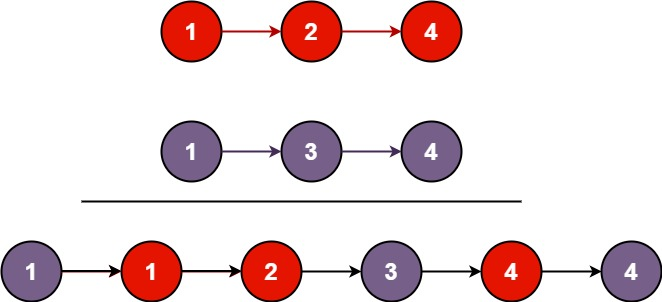# GeetCode Hub

Merge two sorted linked lists and return it as a sorted list. The list should be made by splicing together the nodes of the first two lists.

Example 1:```Input: l1 = [1,2,4], l2 = [1,3,4]
Output: [1,1,2,3,4,4]
```

Example 2:

```Input: l1 = [], l2 = []
Output: []
```

Example 3:

```Input: l1 = [], l2 = 
Output: 
```

Constraints:

• The number of nodes in both lists is in the range `[0, 50]`.
• `-100 <= Node.val <= 100`
• Both `l1` and `l2` are sorted in non-decreasing order.

/** * Definition for singly-linked list. * public class ListNode { * int val; * ListNode next; * ListNode() {} * ListNode(int val) { this.val = val; } * ListNode(int val, ListNode next) { this.val = val; this.next = next; } * } */ class Solution { public ListNode mergeTwoLists(ListNode l1, ListNode l2) { if(l1 == null && l2 == null){ return null; } if(l2 == null){ return l1; } if(l1 == null){ return l2; } if(l1.val < l2.val){ l1.next = mergeTwoLists(l1.next, l2); return l1; } else{ l2.next = mergeTwoLists(l1,l2.next); return l2; } } }

This question requires an understanding of LinkedList, how we can use the pointer to keep track of our current position and keep appending the smaller element from the list.

Iterative Approach:
Time Complexity: O(m+n) //where m and n are the lengths of linkedlist1 and linkedlist2

Space Complexity: O(1)

Recursive Approach:

Time Complexity: O(m+n) //where m and n are the lengths of linkedlist1 and linkedlist2

Space Complexity: O(m+n) //for system in-memory stack